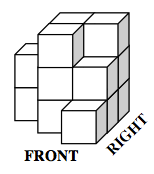### Home > CCG > Chapter 10 > Lesson 10.3.5 > Problem10-183

10-183.

For each mat plan, create an isometric view of the solid.

1.  Back $2$ $3$ $3$ Left $3$ $2$ Right $1$ Front1.  Back Left $1$ $2$ $2$ $1$ Right $1$ $1$ $1$ $1$ $1$ $1$ Front

Use the eTool below to create isometric views.
Click the link to the right for full version. 10-183 HW eTool